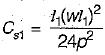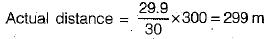Courses

# Linear Measurements - 1

## 10 Questions MCQ Test Mock Test Series for Civil Engineering (CE) GATE 2020 | Linear Measurements - 1

Description
This mock test of Linear Measurements - 1 for Civil Engineering (CE) helps you for every Civil Engineering (CE) entrance exam. This contains 10 Multiple Choice Questions for Civil Engineering (CE) Linear Measurements - 1 (mcq) to study with solutions a complete question bank. The solved questions answers in this Linear Measurements - 1 quiz give you a good mix of easy questions and tough questions. Civil Engineering (CE) students definitely take this Linear Measurements - 1 exercise for a better result in the exam. You can find other Linear Measurements - 1 extra questions, long questions & short questions for Civil Engineering (CE) on EduRev as well by searching above.
QUESTION: 1

### Which of the following instruments is generally used for base line measurements?

Solution:

Invar tapes are used for linear measurement of very high degree precision such as base line measurements cloth or lines tap for rough and subsidiary measurements such as offset.

QUESTION: 2

Solution:
QUESTION: 3

### Which of the following methods of offsets involves less measurement on the ground?

Solution:
QUESTION: 4

The permissible error in chaining for measurement with chain on rough or hilly ground is

Solution:
QUESTION: 5

The correction for sag is

Solution:

Always negative,(Assuming parabola curve),
l1 = length suspended (m)
p = pud applied (kg or N)
w = weight of tape per meter length
wl1 = weight of tape suspended between supports

QUESTION: 6

The maximum tolerance in a 20 m chain is

Solution:

The maximum tolerance in 20 m chain is ±5 mm in 30 m chain is ±8 mm

QUESTION: 7

The correction to be applied to each 30 metre chain length along θ° slope, is

Solution:
QUESTION: 8

A 30 m metric chain is found to be 0.1 m too short throughout the measurement. If the distance measured is recorded as 300 m, then the actual distance measured will be

Solution:

Actual length of chain = 30 - 0.1 = 29.9 mQUESTION: 9

The length of a chain is measured from

Solution:
QUESTION: 10

A surveyor measured the distance between two points on the plan drawn to a scale of 1 cm = 40 m and the result was 468 m. Later he discovered that he used a scale of 1 cm = 20 m. What is the true distance between the two points?

Solution:

Distance between two points measured with scale of 1 cm to 20 cm
= 468/20 = 23.4 cm
Actual scale of the plan is 1 cm = 40 m
∴ True distance between points
= 23.4 x 40 = 936 m